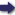(London :  Kegan Paul, Trench, Trübner & Co.,  1910.)

 Tools

## Search this bookPrev Page 47 Next``` CHAPTER LIII. 47 from the middle number, and disregard the remainder which you have got by the division. Divide the middle number by 976. The quotient is the number of com¬ plete adhimdsa months, and the remainder is that which has elapsed of the current adhimdsa month. " Multiply these months by 30, and add the product to the upper number. The sum is the number of the partial lunar days. Let them stand in the upper place, and write the same number in the middle place. Mul¬ tiply it by II, and add thereto 497. Write this sum in the lower place. Then divide the sum by 111,573. Subtract the quotient from the middle number, and dis¬ regard the remainder (which you get by the division). Further, divide the middle number by 703, and the quotient represents the unardtrct days, the remainder the avamas. Subtract the ilnctrdtra days from the upper number. The remainder is the number of civil days." Page 226. This is the ctharganct of the Khctndiakhddyctka. Divid¬ ing the number by 7, the remainder indicates the week¬ day on which the date in question falls. We exemplifv this rule in the case of our gauefe-vear. Application ^ •{ / . ° ° "^ of this The corresponding year of the Sctkakdla is 953. We method to subtract therefrom 587, and get the remainder 366. year. We multiply it by the product of 12 x 30, since the date is without months and days. The product is 131.760, i.e. solar days. We write down this number in three different places. We add 5 to the middle and lower numbers, whereby we get 131,765 in both places. We divide the lower number by 14,945. The quotient is 8, which we sub¬ tract from the middle number, and here we get the remainder 131,757. Then we disregard the remainder in which the division has resulted. Further, we divide the middle number by 976. The quotient 134 represents the number of months. There is besides a remainder of f f f. Multiplying the months by 30, we get the product 4020, which we add to the ```Prev Page 47 Next